# Abstracts

The present work emphasizes the importance of testing hypothesis on homogeneity of covariance matrices from multivariate k populations. The violation of the assumption of the homogeneity of covariance matrices affects the performance of the tests and the coverage probability of the confidence regions. This work intends to apply two tests of homogeneity of covariance and to evaluate type I error rates and power using Monte Carlo simulation in normal populations and robustness in non normal populations. Multivariate Bartlett's test (MBT) and its bootstrap version (MBTB) were used. Different configurations are tested combining sample sizes, number of variates, correlation and number of populations. Results show that the bootstrap test was considered superior to the asymptotic test and robust, since it controls the type error I rate.

Simulation; type I error rate; power, Monte Carlo

O presente trabalho ressalta a importância da aplicação de testes sobre a hipótese de igualdade de matrizes de covariâncias de k populações. A violação da pressuposição da homogeneidade das covariâncias afeta diretamente a qualidade dos testes e a probabilidade de cobertura das regiões de confiança. Por essa razão, neste trabalho propõe-se aplicar dois testes de homogeneidade de covariâncias e avaliar as suas performances mediante uso de simulação Monte Carlo em populações normais e a robustez em situações não-normais avaliando-se o erro tipo I e o poder. Os testes utilizados foram: teste de Bartlett multivariado e a sua versão bootstrap. Foram feitas combinações entre os tamanhos amostrais, número de variáveis, correlação e número de populações. Os resultados obtidos permitiram concluir que o teste de bootstrap foi considerado superior aos assintótico e robusto, controlando o erro tipo I.

Simulação; erro tipo I; poder, Monte Carlo

CIÊNCIAS AGRÁRIAS

Robustness of asymptotic and bootstrap tests for multivariate homogeneity of covariance matrices

Robustez de testes assintóticos e de Bootstrap para homogeneidade de covariâncias em populações multivariadas

Roberta Bessa Veloso SilvaI; Daniel Furtado FerreiraII; Denismar Alves NogueiraIII

IMestre  Departamento de Ciências Exatas/DEX  Universidade Federal de Lavras/UFLA  Cx. P. 3037  37200-000  Lavras, MG  ssaveloso@yahoo.com.br

IIPh.D.  Departamento de Ciências Exatas/DEX  Universidade Federal de Lavras/UFLA  Cx. P. 3037  37200-000  Lavras, MG  Universidade Federal de Lavras/UFLA  Cx. P. 3037  37200-000  Lavras, MG  danielff@ulfa.br; Bolsista CNPq

IIIMestre  Departamento de Ciências Exatas/DEX  Universidade Federal de Lavras/UFLA  Cx. P. 3037  37200-000  Lavras, MG  denisnog@yahoo.com.br

ABSTRACT

The present work emphasizes the importance of testing hypothesis on homogeneity of covariance matrices from multivariate k populations. The violation of the assumption of the homogeneity of covariance matrices affects the performance of the tests and the coverage probability of the confidence regions. This work intends to apply two tests of homogeneity of covariance and to evaluate type I error rates and power using Monte Carlo simulation in normal populations and robustness in non normal populations. Multivariate Bartlett's test (MBT) and its bootstrap version (MBTB) were used. Different configurations are tested combining sample sizes, number of variates, correlation and number of populations. Results show that the bootstrap test was considered superior to the asymptotic test and robust, since it controls the type error I rate.

Index terms: Simulation; type I error rate, power, Monte Carlo.

RESUMO

O presente trabalho ressalta a importância da aplicação de testes sobre a hipótese de igualdade de matrizes de covariâncias de k populações. A violação da pressuposição da homogeneidade das covariâncias afeta diretamente a qualidade dos testes e a probabilidade de cobertura das regiões de confiança. Por essa razão, neste trabalho propõe-se aplicar dois testes de homogeneidade de covariâncias e avaliar as suas performances mediante uso de simulação Monte Carlo em populações normais e a robustez em situações não-normais avaliando-se o erro tipo I e o poder. Os testes utilizados foram: teste de Bartlett multivariado e a sua versão bootstrap. Foram feitas combinações entre os tamanhos amostrais, número de variáveis, correlação e número de populações. Os resultados obtidos permitiram concluir que o teste de bootstrap foi considerado superior aos assintótico e robusto, controlando o erro tipo I.

Termos para indexação: Simulação; erro tipo I; poder, Monte Carlo.

INTRODUCTION

In the multivariate inference, in general, is assumed normality, homogeneity of the covariance matrices and independence of the sample observations. The violation of the assumption of covariance homogeneity has directly effects on tests performance and on the coverage probability of the confidence regions. For this reason tests for the null hypothesis of equality of k populational covariance matrices must be applied. For the specific case of normal samples there are already several usual and reliable tests in the literature.

The researchers in general have the need of testing hypotheses on populational parameters. When testing the hypotheses the researcher takes the risk of making wrong decisions, in other words, to incur in errors. These are called type I and type II errors. The type I error is committed when ones rejects the null hypothesis given that it is true and the probability of incurring in this type error is given by the significance level a (MOOD et al., 1974). It is not possible to control at the same time the probabilities of the two types of error, although the type I error rates can be controlled. The type II error incurs when one accepts the null hypothesis when it is false and the probability associated to that error is b. For any tests of hypotheses or decision rules have acceptable results, they should be planed to minimize the decision errors. This is not a simple task, because for a settled sample size, the attempt to reduce certain type of error is accompanied by the increment of the other. It should be noticed that the type I and type II error rates are inversely proportional (BORGES & FERREIRA, 2002). A balance among these error rates is essential, so that the type II error rate is not excessively increased. Usually, a researcher determines the value a and ignores the values b. As consequence it is easier to incur in a type II than in a type I error. The probability (1  b) of not incurring a type II error is the power of the test, that is the probability of rejecting H0, given it is false. The power of a test (Borges & Ferreira, 2002) is the probability of detecting differences among treatment levels when they really exist.

The homogeneity tests assume multivariate normality. If this assumption was violated these tests are sensitive on the control of the type I error and on the power. They do not differentiate between the lack of normality and possible covariance heterogeneity. The test of equality of k covariance matrices proposed by Bartlett (1954), one of the most used, is strongly affected when the assumption of multivariate normality is violated. The bootstrap tests have been suggested in the literature as an alternative to accomplish inference in the most varied situations. In the specific case of the covariance matrices comparisons, the tests were already successfully used, however, in very restricted situations.

This article aimed to propose a bootstrap version of the multivariate Bartlett test for the equality of covariance matrices and to compare its performance with the asymptotic approach, evaluating the power and the type I error rates in normal and non-normal situations, where this last case was used to check the robustness.

METHODOLOGY

Two tests were considered in this article: the multivariate Bartlett's test (MBT) for equality of covariance matrices and its respective bootstrap version (MBTB).

Let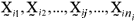be a random sample of size ni from the ith population, whereis the random p-dimensional vector from the jth sample unit and ith population, considering i = 1, 2, ¼, k and j = 1, 2, ¼, ni. Let the ith populational mean vector be, the covariance matrix be Si (p x p) and n =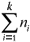.

The hypothesis of interest is given by:The multivariate test of Bartlett for covariance matrices

Under the alternative hypothesis, H1, from equation ( 1) and under multivariate normality, the likelihood function is given by: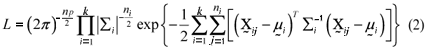The likelihood function (2) was maximized. Thus, the maximum likelihood estimators of the ith populational mean vectors and covariance matrices were: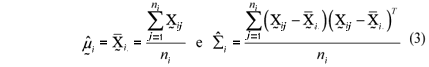for i = 1, 2, ¼, k.

Under the null hypothesis H0 and multivariate normality the likelihood function is:Proceeding in the same previous way the populational mean vectorsestimators were identical to those of the equations (3) and the common populational covariance matrix (S) estimator was:It is convenient to substitute the covariance matrices maximum likelihood estimators by the unbiased estimators given by: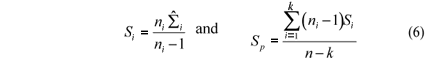The modified likelihood ratio statistic isAs -2 ln L is asymptotically distributed as c2 (MOOD et al., 1974), the test statistic of the MBT is given by:where v = (k - 1) p (p + 1)/2. The degrees of freedom n were obtained comparing the dimension of the unrestricted likelihood function [pk + p(p + 1)k/2] with the dimension of the restricted likelihood function [pk + p(p + 1)/2]. Bartlett (1954) proposed a correction to improve the asymptotic properties of the approach. The modified test statistic is: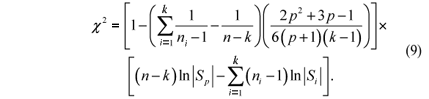Bootstrap test

A bootstrap version of the asymptotic test presented in section 2.1 was proposed. The null hypothesis H0 was imposed combining the k multivariate samples in a common sample of size n =with p-dimensional observations. The original observations were replaced by deviations. According to Johnson & Wichern (1998), those deviations do not change the ith sample variability, since they represented translations in the original scale given by the sample mean. That precaution was taken to avoid those potential differences in the population means could be responsible for significantly differences in the results of bootstrap test for comparing covariance matrices.

The multivariate bootstrap test of Bartlett for comparing covariance matrices was denoted MBTB. The basic ideas is to impose the null hypothesis H0 combining the samples into one, to resample with replacement the combined sample reproducing k new samples of size ni, i = 1,2, ¼, k, preserving the dimension and then computing the test statistics using equation (9). The results obtained in B resamples were compared with the statistic values obtained in the original samples. If the proportion of values of the bootstrap distribution that overcame the test original values was inferior to the nominal significance level a the hypothesis H0 should be rejected. The algorithm for the bootstrap test, MBTB, is given by:

1.determine the c2 statistic in the original sample using (9);

2.create the setW =using the p-variate residual deviations from all sample observations;

3.resample W with replacement, reproducing new k samples, where the elements were deviations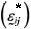random selected from W, given by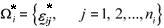for i = 1, 2, ¼, k;

4.restimate the bootstrap covariance matrixfrom the set;

5.estimate the statistic (9) and denote it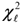for theth bootstrap resampling, where= 1, 2, ¼, B;

6.replicate B times the steps 3 to 5;

7.determine the boostrap p-values by

where I() is an indicator function.

Monte Carlo simulations

The performance of the two tests was evaluated by computational Monte Carlo simulation. The type I error rate and power were recorded to determine the quality of the performance of the tests. Two different situations were also considered for evaluating the tests. First, it was considered the multivariate normal distribution and then it was simulated multivariate distributions using a linear transformation of random vectors formed by uniform and gamma independently distributed variates. In the last situation the evaluation of the robustness of the two tests for the covariances comparison was accomplished measuring the type I error rates.

For evaluating the tests performance simulations were accomplished considering different numbers of population (k), sample sizes (ni) and number of variates (p). It still was considered three p-dimensional distributions obtained from linear transformations of normal, gamma and uniform univariate variates. Those parameters (k, ni and p) were combined in factorial combination to simulated sample data from those three distributions.

The k values were 2, 5 and 10, the number of variates p was 2, 3, 5 and 10 and the sample sizes varying from 5 to 100. It was made 600 simulations of each configuration, of each distribution and under and H0 and H1. The MBT and MBTB were applied in each case. Two nominal significance levels a (0.01 and 0.05) were adopted. The observed p-values were confronted with the nominal significance levels a. The proportion of simulations results that the test's p values were inferior or equal to the nominal level was computed. Under the null hypothesis H0 this proportion refers to the type I error rates and under H1, to the power.

Non-normal situations were used to evaluate the robustness of the tests as in the control of the type I error rates as in the power. The normal distribution was used in simulations to propitiate an ideal condition that served as reference for the other non-normal distributions. The IML procedure of SAS® (SAS INSTITUTE, 2000) was used in this work. The following algorithm was adopted for simulating the jth observation from the ith sample:

a) it was generated realizations of p variates from each one of the three distributions. To generate samples from the univariate normal distribution the function rannor(seed) of the SAS®(SAS INSTITUTE, 2000) was used, where seed represents the initial value of the process of generation of random numbers; from the uniform distribution, the function ranuni(seed); and from the gamma, rangam (seed, l). The seed value was settled as zero, so that the SAS system could use an initial value from the time of day. The l parameter from the gamma distribution was settled in 1.5, to obtain a unimodal and right-skewed distribution;

b) the realizations of the independent and identically distributed (i.i.d) variates (Zr) were used to compose the vector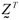= [Z1, Z2, ¼, Zp];;

c) it was considered a single structure for the covariance matrix for ith population given by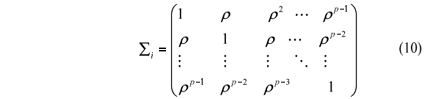For evaluating the type I error rates it was simulated k populations with the same covariance matrix Si = S, " i = 1, 2, ¼, k, i.e., it was performed simulations under the null hypothesis H0 of (1). For the power, the covariance matrices S2, S3, ¼, Sk were determined as a multiple of S1, considering a criterion that depends on a coefficientfor determining a degree of heterogeneity of the covariance matrices. For that, the matrix S1 was settled by the expression (10) and the other covariance matrices were given byThe criterion used to determinewas based on the generalized variances of S1 as follow. First it was settled the value of d, given byas 2, 4, 8 and 16. Then, the auxiliary quantity d was calculated byand finally the quantity d* was obtained by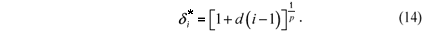According to the previously defined quantities, the parameter d represents the ratio between the largest and smallest generalized variances and the consecutive differences among the ratios of generalized variances between each population and the first are d. Then, the p values were settled in 0, 0.1, 0.5 and 0.9.

d) the Cholesky factor of each covariance matrix was calculated by

where the Fi matrix is the Cholesky factor of Si and was obtained using the function root (Si) from the SAS® System (SAS INSTITUTE, 2000), i = 1, 2, ¼, k;

e) the p-variate random vectorfrom the ith population and jth sample unit was generated by the linear transformation given by

where, without loss of generality, the mean vectorwas considered equal to a p dimensional vector of zeros;

f) the steps (a), (b) and (e) were iterated until a sample of size n =was simulated.

RESULTS AND DISCUSSION

In the sequence the main results will be presented for the type I error rates and power of the two tests studied in this article. Two different situations were considered where samples from normal and non-normal (uniform and gamma) populations were drawn. Also, two nominal significance levels (5% and 1%) were considerate.

Type I error rates under normal distribution

In Table 1 the type I error rates of the MBT and MBTB are presented under normality and considering k = 2 populations for different values of p, p and ni. The 95% confidence interval for the nominal significance level of 5% is [0.034; 0.071]. The results of the type I error rates were confronted with this interval.

The MBT controlled the type I error rates in almost all of simulated situations, except for some situations with p = 3 for the sample sizes n1 = n2 = 5, for p = 0.1; n1 = 5; and n2 = 20 for p =0 and p = 0.9; n1 = 5 and n2 = 60 for p = 0 and p = 0.5; n1 = 7 and n2 = 20 for p = 0.1. For these situations MBT did not control the type I error rates and presented superior rates to the nominal significance level of 5%, and it is considered liberal (results not presented). A test is considered liberal when the type I error rates are significantly superior to the nominal significance level.

The correlation structure had small or any influence in the type I error rates of MBT and of MBTB (Table 1). For instance, considering n1 = n2 = 5 one can realize that the changes in the correlation coefficient did not alter the type I error rates significantly. Therefore, for the other sample sizes the results were presented only for p = 0.5.

The MBTB, in general, was conservative test for small samples, presenting in some situations null type I error rates (Table 1). A test is conservative when the type I error rates are significantly inferior to the nominal significant level. There was a tendency of MBTB performance to improve as the sample sizes increase, considering that it presented type I error rates not significantly different from the nominal level of 5%. It was observed that the increase of the number of variates also affected the multivariate bootstrap test (MBTB) performance, turning this test more conservative in opposition to the effect caused in the MBT.

For k = 5 populations MBT did not maintain the same performance of the situation of k = 2, once it was, in general, more liberal. The liberality increased as increase the number of variates, for samples of different and intermediate sizes in the two populations. MBTB tended to be conservative for smaller samples. For sample sizes (ni) at least equal to 30, the type I error rates were similar to the nominal significance level of a = 5%, except for p = 10.

The type I error rates of the two tests for k = 10 populations showed that there were improvements as the number of populations k increased from 2 to 10 (Table 1). The MBT had the best performance. These results for populations showed the same tendencies already discussed for k = 2 and k = 5. It is convenient to point out that there is no remarkable correlation effect of the type I error rates in the different tests (Table 1). The MBTB was shown conservative for p = 10, while MBT controlled the type I error rates.

The type I error rates were evaluated also for the nominal significance level of 1% (a), k = 2 populations and some representative situations of sample size ni. The 95% confidence interval to the nominal significance level a = 1% for the type I error rates is [0.004; 0.022]. Usually, the same tendency was verified in the results when compared to the situation of a = 5 . The type I error rates of the MBT did not present results significantly different from the nominal level. For large sample sizes (n1 = n2 = 60) and number of variates (p = 10) , the MBTB becomes conservative (results not presented).

For k = 5 populations and a = 1%, it was observed that the type I error rates of the two tests maintained the performance described previously. It can be emphasized the effect of the number of variates, mainly when p is large compared to the minimum of ni among all of the k populations. With the increase of the MBT and the MBTB became, in almost all cases, conservative.

Type I error rates for non-normal distributions (uniform and gamma)

Type I error rates for the two tests are presented in Table 2, considering some specific cases of sample sizes, p and p for a settled number of populations (k = 2) and for the uniform distribution. The MBT and the MBTB became more conservative than these same tests in the normal case. The MBTB was conservative for small samples and tended to present error rates not significantly different from the nominal significance level a = 5% , for large samples, except for p > 5.

For larger number of variates, the asymptotic test (MBT) tended to become conservative, with type I error rates significantly (p < 0,05) smaller to the nominal significance level. In the bootstrap version (results not presented), the simulated error rates were almost always no significantly different from the nominal level of . Generally, the type I error rates were controlled, since the observed values always were equal or smaller than the nominal significance level.

Type I error rates for MBT and MBTB are presented in Table 3, considering simulations accomplished from gamma distribution with shape parameter l = 1.5 and k = 2 populations. It can be noticed that the asymptotic MBT was liberal for all the sample sizes simulated. As larger the sample sizes most liberal were the test. That shows that this test is not robust to the normality violation and that seemingly it is more affected for skewness deviations. These results are similar to those of Zhang & Boos (1992). A surprising result was the increasing of the test liberality with the increase of the sample sizes, since it is expected the opposite.

The bootstrap version (MBTB) was conservative in small samples or had exact size for a = 5%. That transition between liberal to conservative when one changes the asymptotic test for the bootstrap version is desirable, although it is not ideal. There was a control of the type I error rate, although these rates are significantly smaller than the nominal significant level. Considering large samples and high dimensions, the MBTB tended to be conservative.

The analysis of the results for the type I error rates of the tests for a = 5% and k = 5 populations, considering the p-multidimensional gamma distribution and different sample sizes showed that there was the same response pattern of the situation of k = 2 populations. The difference is an accentuation of the liberality of the asymptotic test (MBT). The MBTB was always conservative or of exact size a = 5% (results not presented).

For k = 10 populations the same response pattern for and was observed, except for an accentuation of the liberality of the MBT. Usually, it can be seen that the asymptotic test (MBT) is not robust considering the type I error rates for gamma distributions (MOOD et al., 1974). The bootstrap test (MBTB) controlled the type I error rates by keeping the empiric rates equal or smaller to the nominal significance level of 5%. Thus, the MBTB can be considered a robust test in the control of the type I error rates.

Power of the tests under normality

For the power study of the tests the correlation was settled in p = 0.5. This strategy was considered due to the results of the type I error rates have not been affected by the correlation structure. Although, some cases were simulated to evaluate the power considering different correlations (results not presented) and the same response pattern observed under the null hypothesis was verified. Thus, the results of power for the two tests considering , different sample sizes and dimensions, a = 5%, k = 2 and sampling from normal populations are presented in Table 4.

Considering d = 2, a situation of small difference among the covariance matrices, the values of power of the tests were small, since they were equal or smaller than the nominal significance level a = 5% , mainly with small sample sizes. As the sample sizes increase, the power of the tests also increases (Figure 1). These results are expected, since the tests are, generally, conservative for small samples.

An important aspect is how to associate the sample sizes with the populations, when the sample sizes are different. It can always associate the smallest sample size with the population of smaller generalized variance, the second smallest sample size with the population of second smaller generalized variance and so on. The generalized variance can be expressed by the determinant of the sample covariance matrix, which reduces to the sample variance for the case of a single variate (p = 1). This determinant is called sample generalized variance.

The association between the samples sizes and the populations also can be made to verify the effect in the power of the tests. For the type I error rate, any mention to that fact was made because the populational covariances were the same. This effect is important to be evaluated, mainly when the researcher intends to accomplish unbalanced experiments. In real situation, it is impossible for the researcher to do any type of association like that, because the covariance matrices (Si) are not known. However, it is important to study this effect, mainly for evaluating if there are interactions between heterogeneity and sample sizes associations of the populations.

Cases considering d = 2 and changes in the sample sizes from (n1 = 15, n2 = 60) to (n1 = 60, n2 = 15) and from (n1 = 15, n2 = 40) to (n1 = 40, n2 = 15) were evaluated. The response pattern was basically the same, i.e., the relative performance of the tests was the same independently of the association between sample sizes and the values of the populational generalized variances. The MBT was slightly superior to the MBTB.

Considering d = 4, k = 2, a = 5% and p = 2 under multivariate normality, the same response pattern was observed for the power of the two tests. The associations of the smaller samples with the populations of smaller generalized variances resulted in a lower power of the tests than the cases that the largest samples were associated to the populations of smaller generalized variances.

The power relative response pattern of the tests for k = 5 and k = 10 populations, considering normal populations, with com p = 2 and d = 2 or 16 is exactly the same of the cases for k = 2. It can notice that as the number of populations increases one can expect a decrease in the power of the tests. Generally, the magnitude of that reduction is small and less than the effect of the increase in the number of variates. It is also convenient to point out that this effect is pronounced for samples of intermediate sizes (5 < ni < 20). A great differentiation among tests was observed with large d (d = 16) and small sample sizes.

Power of the tests under non-normality

The power of the tests was evaluated under atypical conditions and for that two distributions (uniform and gamma) were considered. The results allowed detecting that the bootstrap test had best performance compared to the counterpart asymptotic tests in larger samples. In this case, the MBTB presented power of 40% under uniform distribution (d = 2) and 29.5% under normal distribution.

The MBT in small samples performed better than MBTB and in intermediate sample sizes, it performed worst. There was little differentiation of the tests in the case of large differences among the populational generalized variances (d = 8).

The results under uniform distribution considering k = 10 populations, d =2 and 8, p = 2 variates and different sample sizes allowed conclude that there was a sensitive power reduction of MBT. The MBTB suffered a small reduction in the power, when the number of populations changes from k = 2 to k = 10.

The asymptotic test had larger power, as already expected, considering the gamma distribution in the case of (Table 5). This larger power is not real, because the size of the test is larger than the nominal significance level under the null hypothesis.

The power of the MBTB was small for d = 2 and small sample sizes. For larger samples (n1 = n2 = 60), the power was 12.2% in gamma and 29.5% in normal distributions, respectively. There was reduction of the power from normal to gamma distributions, but it was the opposed that occurred in the uniform distribution.

When the difference among the covariance matrices is large (d > 2), the response pattern observed for d = 2 is maintained, but occurring only an expected increase in the power. As type I error rates of the asymptotic test tend to increase with the increase of the sample size. Then, the asymptotic test, which theoretically should be recommended for larger samples, cannot be done in gamma distributions. The results of power, observed for showed that the differences between the asymptotic and bootstrap tests amplified, mainly considering d = 2. However the general comparative response pattern of the tests is the same related for the k = 2 populations case.

CONCLUSIONS

This Monte Carlo simulations applied in this article allowed that one concluded that the bootstrap multivariate test (MBTB) was considered a superior and robust test when compared to the asymptotic alternative (MBT), because this test controlled the type I error rates.

BIBLIOGRAPHIC REFERENCES

(Received in june 2, 2005 and approved in june 6, 2006)

• BARTLETT, M. S. A note on the multiplying factors for various c2 approximations. Journal of Royal Statistical Society B, London, v. 16, n. 2, p. 296-298, 1954.
• BORGES, L. C.; FERREIRA, D. F. Poder e taxas de erro tipo I dos testes Scott-Knott, Tukey e Student-Newman-Keuls sob distribuições normal e não-normais dos resíduos 2002. 94 p. Dissertação (Mestrado em Estatística e Experimentação Agropecuária) Universidade Federal de Lavras, Lavras, 2002.
• JOHNSON, R. A.; WICHERN, D. W. Applied multivariate statistical analysis 4. ed. New York: Prentice Hall, 1998. 816 p.
• MOOD, A. M.; GRAYBILL, F. A.; BOES, D. C. Introduction to theory of statistics 3. ed. New York: Wiley & Sons, 1974. 842 p.
• SAS INSTITUTE. SAS for Windows, release 8 Cary, 2000.
• ZHANG, J. I.; BOOS, D. D. Bootstrap critical values for testing homogeneity of covariance matrices. Journal of the American Statistical Association, Alexandria, v. 87, n. 418, p. 425-429, June 1992.

# Publication Dates

• Publication in this collection
12 Mar 2008
• Date of issue
Feb 2008

• Accepted
06 June 2006